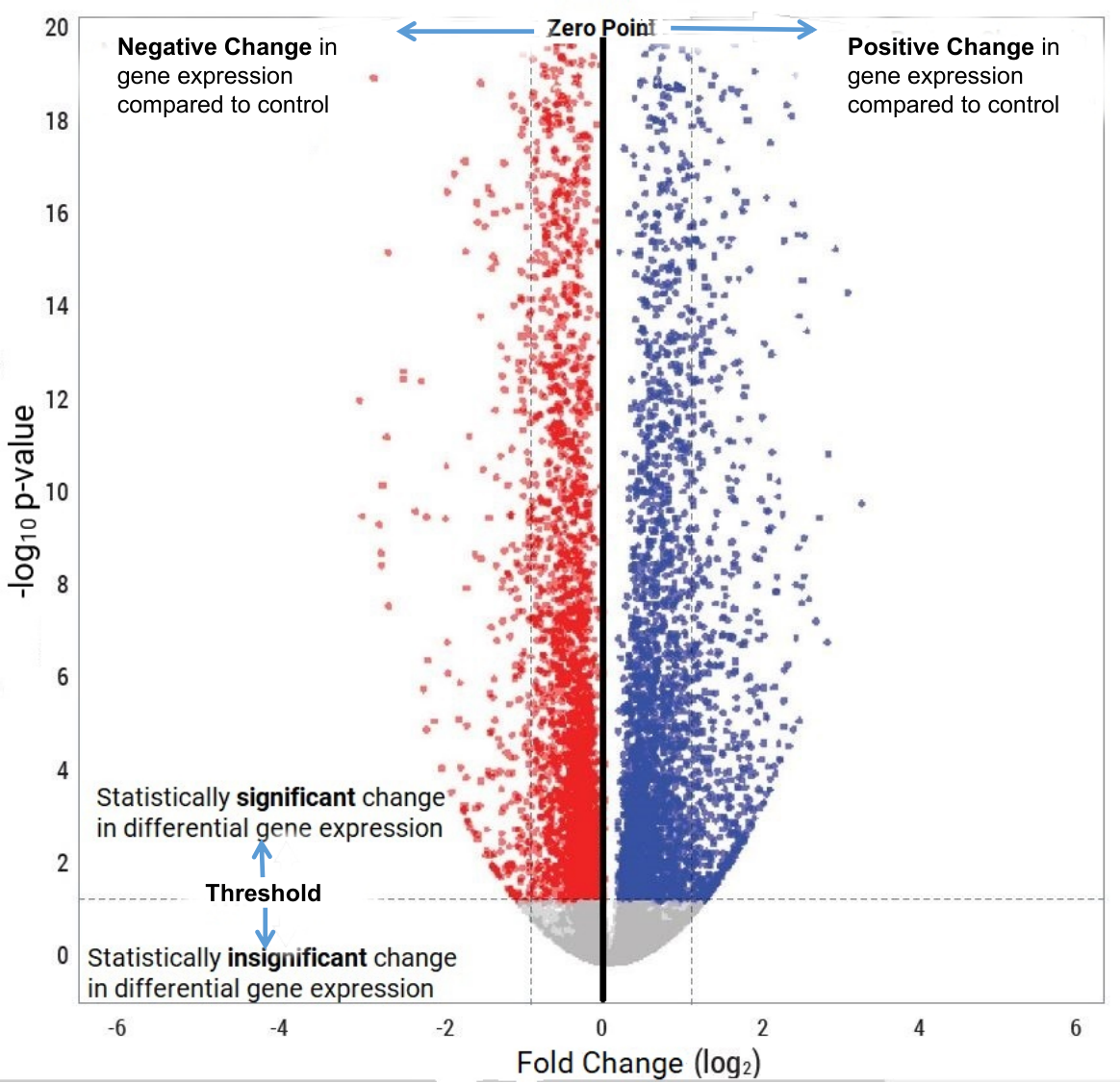A volcano plot is a graphical representation used to visualize the results of statistical tests applied to high-dimensional biological data, such as gene expression data. The primary purpose of a volcano plot is to highlight genes that are significantly differentially expressed between two experimental conditions. It achieves this by simultaneously displaying both the statistical significance (p-value) and the biological relevance (fold change) of each data point.

Key Components of a Volcano Plot:

Fold Change (FC): The x-axis of the volcano plot represents the fold change in gene  expression between two conditions. Fold change quantifies how much the expression of a gene has increased or decreased. A point located far to the left or right of the plot indicates a substantial fold increase or decrease. The data is plotted in log2 scale so a value of 1 indicates 2 fold increase

Statistical Significance (p-value): The y-axis of the volcano plot represents the statistical significance of the differential expression. This is typically measured by a statistical test like t-test or ANOVA. Data points with low p-values (usually below a predetermined threshold, such as 0.05) are considered statistically significant.

Interpreting a Volcano Plot:

A volcano plot can be divided into four regions:

Upper Right Quadrant: Genes in this region have a significant fold increase and are statistically significant. These are the most interesting candidates for further investigation as they are highly likely to be differentially expressed between the conditions.

Upper Left Quadrant: Points in this region represent genes with a significant fold decrease but not statistically significant. They may still be biologically relevant, but more data or validation is needed to confirm their significance.

Lower Right Quadrant: Genes in this region are statistically significant but do not exhibit a substantial fold change. These may be of limited biological importance but could still warrant investigation, especially if they are part of a larger regulatory network.

Lower Left Quadrant: This region contains genes that are neither statistically significant nor biologically relevant. They are typically considered noise and are less likely to be pursued further.